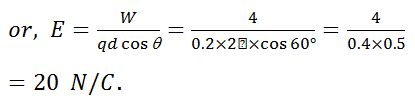# There is an electric field E in x-direction. If the work done on moving a charge of 0.2 C through a distance of 2 m along a line making an angle 60° with x-axis is 4 J, then what is the value of E?         (a)    3 N/C         (b)    4 N/C         (c)    5 N/C         (d)    20 N/C

10 years ago

(d)

Charge (q) = 0.2 C; Distance (d) = 2m; Angle

q = 60° and work done (W) = 4J.

Work done in moving the charge (W)3 years ago
hello student,
Work done,W=F.r=Frcosθ=qErcosθ
or1=0.2×E×2cos60
orE=0.2×2cos601​=1/0.2×2(1/2)​=5N/C

hope it helps
thankyouYash Chourasiya
3 years ago
Dear Student

We Know that
F = qE

W = qEΔlcosθ
W = qE2 cos(60)
4 = 0.2E*2*(1/2)
E = 20 NC-1